# To improper fraction

Change mixed number to improper fraction

a) 1 2/15

b) -2 15/17

a =  1.1333
b =  -2.8824

### Step-by-step explanation:

$b=-2\frac{15}{17}=-\frac{49}{17}=-2\frac{15}{17}=-2.8824$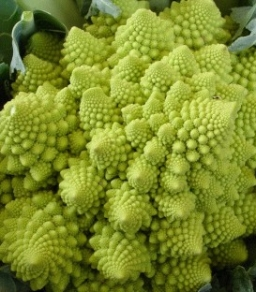Did you find an error or inaccuracy? Feel free to write us. Thank you!

Showing 1 comment:Math student
a fish vendor sells  5/7 kilos of tilapia for 73.50.  if you will buy 2 1/7 kilos of tilapia, how much will it cost?

3 years ago  3 LikesTips to related online calculators
Need help to calculate sum, simplify or multiply fractions? Try our fraction calculator.

## Related math problems and questions:

• The fish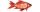The fish vendor sells 1/7 kilos of bangus for 66.2 euro. If you will buy 9 2/8 kilos of bangus, how much will cost?
• Fractions and mixed numerals(a) Convert the following mixed numbers to improper fractions. i. 3 5/8 ii. 7 7/6 (b) Convert the following improper fraction to mixed number. i. 13/4 ii. 78/5 (c) Simplify these fractions to their lowest terms. i. 36/42 ii. 27/45 2. evaluate following ex
• Land cost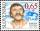The land is worth 33295 CZK. 1st owner has 5/15, 2nd owner has 4/15, 3rd owner has 1/5 4th owner has 1/5. How much CZK each of them will receive?
• Mixed2improperWrite the mixed number as an improper fraction. 166 2/3
• 5 2/55 2/5 hours a week  mathematics,  3 3/4 hours a week   Natural sciences, 4 3/8 hours a week  Technology . how many hours does  he spend on social sciences if he spend 17 1/2 hours a week for the four subject?
• Mixed numbersRewrite mixed numbers, so the fractions have the same denominator: 5 1/5 - 2 2/3
• Evaluate 17Evaluate 2x+6y when x=- 4/5 and y=1/3. Write your answer as a fraction or mixed number in simplest form.
• Blueberries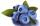Adéla sells blueberries for 50 CZK/1 l, Zdeněk sells 1 kg of blueberries for 75 CZK. Which of them sells irritants if 1 liter of blueberries weighs 650 g?
• The sum 12The sum of 3 mixed numbers is 20 13/15. two of the numbers are 6 1/3 and 7 5/6. what is the third number?
• Fraction of a NumberIf 1/2 of 1/3 of 1/4 of 1/5 of a number is 2.5. what is the number?
• Simplest form of a fractionWhich one of the following fraction after reducing in simplest form is not equal to 3/2? a) 15/20 b) 12/8 c) 27/18 d) 6/4
• RatiosReduce the numbers: 50 in a 1:2 ratio 111 at a ratio of 2:3 70 at 10:50 560 at a ratio of 3:8
• RegroupingSubtract mixed number with regrouping: 11 17/20- 6 19/20
• The fence 2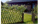A certain type of fencing sells for \$9 a yard. How much will 10 2/3 yards of fencing cost?
• Between two mixed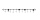What is the rational number between 2 1/4 and 2 4/5?
• Simplify 3Simplify mixed numerals expression: 8 1/4- 3 2/5 - (2 1/3 - 1/4) Show your solution.
• Company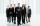After increasing the number of employees by 15% company has 253 employees. How many employees take?